HOMEWORK SOLUTION

HW #5

GEOTECHNICAL ENGINEERING I

(Question no. 5.7b, 5.10, 5.13a, 5.14a, & 5.19 ..Al-Khafaji textbook)5.7b     Compute the seepage in SI unit (m3/day/m), assume that k=10-5 cm/s

Solution:

From the figure 1. obtained       Ne=15             DH=60-45=15 ft

Nf=7

k=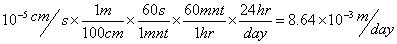DH=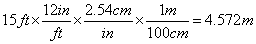q/L=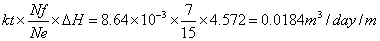…..ans.

5.10     Compute the seepage in m3/day/m, assume k=7x10-4 cm/s

Solution:

From the figure 2. obtained       Ne=19             DH=20 ft

Nf=6

k=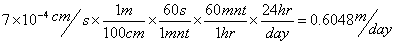DH=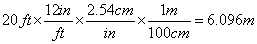q/L(1 side)=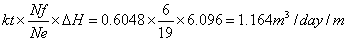for both side.

q/L= 2 x q/L(1 side)=2 x 1.164 = 2.328 m3/day/m …………. Ans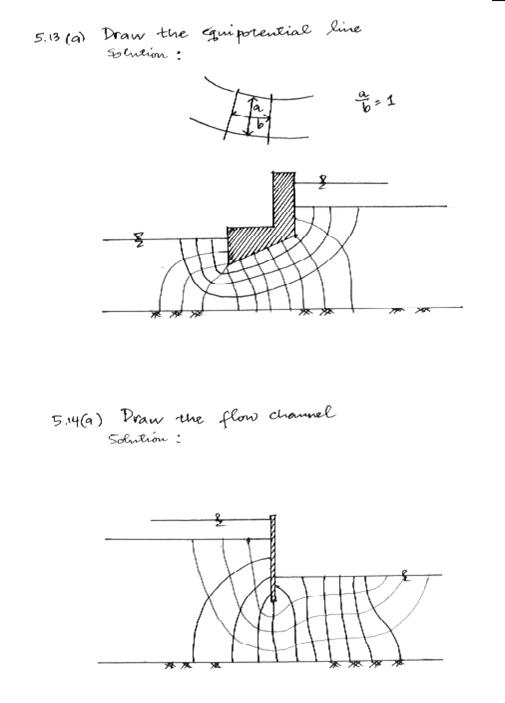5.19          Determine the critical hydraulic gradient and factor of safety against piping for the curvilinear square designated number 10 in the dam profile shown in figure 5.19. Assume that the soil has a specific gravity of solids of 2.65 and void ratio of 0.75.

Solution:

gsat = (Gs+e) gw/(1+e) = (2.65+0.75) 62.4/(1+0.75) = 121.23 lb/ft3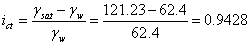………..ans

ic is the critical hydraulic gradient.

Assuming L (square number 10) = 5 ft

Dh = 10/10 = 1 ft/drop

i piping = Dh/L = 1/5 = 0.2

Factor safety = i critical/ i piping = 0.9428/0.2 = 4.7 ~ 5